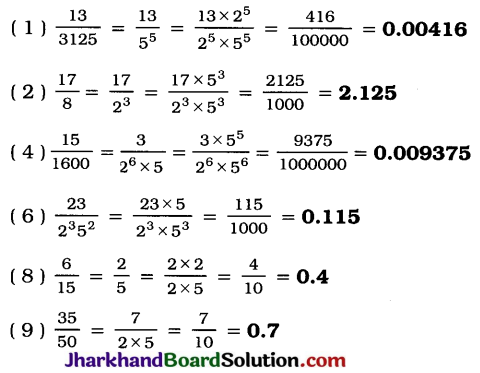# JAC Class 10 Maths Solutions Chapter 1 Real Numbers Ex 1.4

Jharkhand Board JAC Class 10 Maths Solutions Chapter 1 Real Numbers Ex 1.4 Textbook Exercise Questions and Answers.

## JAC Board Class 10 Maths Solutions Chapter 1 Real Numbers Exercise 1.4

Question 1.
Without actually performing the long division, state whether the following rational numbers will have a terminating decimal expansion or a non-terminating repeating decimal expansion:
1. $$\frac{13}{3125}$$
2. $$\frac{17}{8}$$
3. $$\frac{64}{455}$$
4. $$\frac{15}{1600}$$
5. $$\frac{29}{343}$$
6. $$\frac{23}{2^3 5^2}$$
7. $$\frac{129}{2^2 5^7 7^5}$$
8. $$\frac{6}{15}$$
9. $$\frac{35}{50}$$
10. $$\frac{77}{210}$$
Solution:
1. $$\frac{13}{3125}$$
Here, the denominator q(55) is of the form 2n5m with n = 0 and m = 5.
Hence, the decimal expansion of $$\frac{13}{3125}$$ is terminating.

2. $$\frac{17}{8}$$ = $$\frac{17}{2^3}$$
Here, the denominator q(23) is of the form 2n5m with n = 3 and m = 0.
Hence, the decimal expansion of $$\frac{17}{8}$$ terminating.

3. $$\frac{64}{455}=\frac{64}{5 \times 7 \times 13}$$
Here, the denominator q(5 × 7 × 13) is not of the form 2n5m, where n and m are non-negative integers.
Hence, the decimal expansion of $$\frac{64}{455}$$ is non-terminating repeating.

4. $$\frac{15}{1600}=\frac{3}{320}=\frac{3}{2^6 \times 5^1}$$
Here, the denominator q(26 × 51) is of the form 2n5m with n = 6 and m = 1.
Hence, the decimal expansion of $$\frac{15}{1600}$$ is terminating.

5. $$\frac{29}{343}=\frac{29}{7^3}$$
Here, the denominator q(73) is not of the form 2n5m, where n and m are non-negative integers.
Hence, the decimal expansion of $$\frac{29}{343}$$ is non-terminating repeating.

6. $$\frac{23}{2^3 5^2}$$
Here, the denominator q(2352) is of the form 2n5m with n = 3 and m = 2.
Hence, the decimal expansion of $$\frac{23}{2^3 5^2}$$ is terminating.

7. $$\frac{129}{2^2 5^7 7^5}$$
Here, the denominator q (22 × 57 × 75) is not of the form 2n5m, where n and m are non-negative integers. Hence, the decimal expansion of $$\frac{129}{2^2 5^7 7^5}$$ is non-terminating repeating.

8. $$\frac{6}{15}=\frac{2 \times 3}{3 \times 5}=\frac{2}{5^1}$$
Here, the denominator q(51) is of the form 2n5m with n = 0 and m = 1.
Hence, the decimal expansion of $$\frac{6}{15}$$ is terminating.

9. $$\frac{35}{50}=\frac{5 \times 7}{2 \times 5 \times 5}=\frac{7}{2^1 \times 5^1}$$
Here, the denominator q(21 × 51) is of the form 2n5m with n = 1 and m = 1.
Hence, the decimal expansion of $$\frac{35}{50}$$ is terminating.

10. $$\frac{77}{210}=\frac{7 \times 11}{2 \times 3 \times 5 \times 7}=\frac{11}{2 \times 3 \times 5}$$
Here, the denominator q(2 × 3 × 5) is not of the form 22n5m, where n and m are non-negative integers.
Hence, the decimal expansion of $$\frac{77}{210}$$ is non-terminating repeating.Question 2.
Write down the decimal expansions of those rational numbers in Question 1 above which have terminating decimal expansions.
Solution:Question 3.
The following real numbers have decimal expansions as given below. In each case, decide whether they are rational or not. If they are rational, and of the form $$\frac{p}{q}$$, what can you say about the prime factors of q?
1. 43.123456789
2. 0.120120012000120000…
3. $$43 . \overline{123456789}$$
Solution:
1. The given number 43.123456789 is a rational number as its decimal expansion is terminating
Since the decimal expansion of the given number is terminating, in the $$\frac{p}{q}$$ form of the number, the prime factors of q will only be 2 or 5 or both. (Theorem 1.5)

2. The given number 0.120120012000120000…. is an irrational number as its decimal expansion is non-terminating non-repeating.

3. The given number $$43 . \overline{123456789}$$ is a rational number as its decimal expansion is an non-terminating repeating.
Since the decimal expansion of the given number is non-terminating repeating, in the $$\frac{p}{q}$$ form of the number, the prime factors of q will contain at least one prime other than 2 and 5. (Converse of Theorem 1.7)